# 有机化学考证考题（判断题）

## 有机化学考证考前练习题

1、

• A .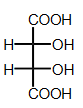• B .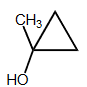• C .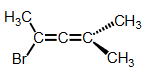• D .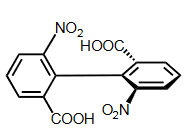2、

• A .• B .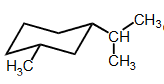• C .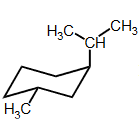• D .3、

• A . 2-甲基丙烯
• B . 丙烯
• C . 2,3-二甲基-1-丁烯
• D . 3-甲基-1-丁炔

4、

• A . 环己烯
• B . 甲基环戊烷
• C . 3,4-二甲基-3-己烯
• D . 1-丁烯

5、

• A . 正戊烷
• B . 氯代丙烷
• C . 丁醛
• D . 丁醇

6、

• A . 乙醇
• B . 对硝基苯甲酸
• C . 苯甲酸
• D . 苯酚

7、

• A . 乙烯
• B . 丙烯
• C . 氯乙烯
• D . 溴乙烯

8、

• A . a>c>d>b
• B . c>a>d>b
• C . c>d>a>b
• D . b>a>d>c

9、

• A . (1)和（4）
• B . (2)和（5）
• C . （3）和（6）
• D . （1）和（6）

10、

• A . 丙醇
• B . 正丁醇
• C . 2-氯乙烷

11、

• A . 1-丁醇
• B . 1,3-丙二醇
• C . 乙醚

12、

• A . C2H5F
• B . CH2=CHCl
• C . Cl2C=CCl2

13、

• A . CH3CH2CH=CH2
• B . 顺-2-丁烯
• C . 反-2-丁烯

14、

• A .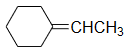• B .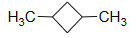• C .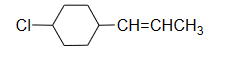15、

• A .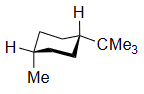• B .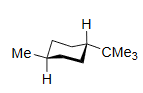• C .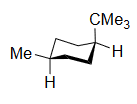16、

• A . CH3CH=CHCH=CH2
• B . CH3CH=C=CHCH3
• C . CH2=CH-CH2-CH=CH3

17、

• A .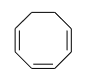• B .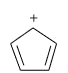• C .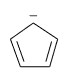18、

• A . 对硝基苯甲酸
• B . 苯甲酸
• C . 苯酚

19、

• A . 苯胺
• B . 乙胺
• C . 氨

20、

• A . Me3C+
• B .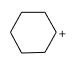• C .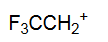21、

• A .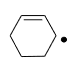• B .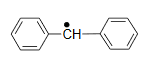• C .22、

• A . CH3COO-
• B . C6H5O-
• C . C2H5O-

23、

• A . 丙烯
• B . 乙烯
• C . 三氟甲基乙烯

24、

• A . 苯
• B . 苯甲酸
• C . 苯甲醚

25、

• A .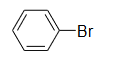• B .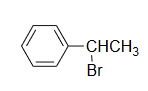• C .26、

• A . 反应速率与亲核试剂浓度无关
• B . 反应物有多种重排产物
• C . 空间位阻影响反应时间

27、

• A .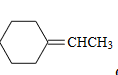• B .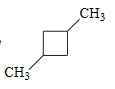• C .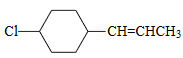• D .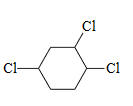28、

• A .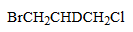• B .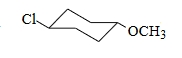• C .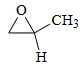• D .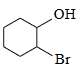29、
1，2-二甲基环己烷最稳定的构象是（）。
• A .• B .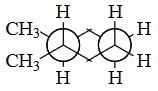• C .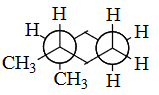30、

• A .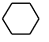• B .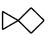• C .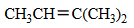• D .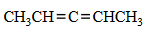31、

• A . CH2CHCl
• B . CH3CCH
• C . CH3CCCH3
• D . CH3CHCH2

32、

• A .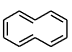• B .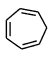• C .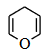• D .33、

• A . 甲醇
• B . 正丙醇
• C . 异丙醇
• D . 叔丁醇

34、

• A . 诱导，p-π,π-π共轭
• B . 诱导，π-π共轭，σ-π超共轭
• C . π-π共轭，σ-π超共轭
• D . 诱导，p-π共轭,σ-π超共轭

35、

• A . ①>②>③
• B . ②>①>③
• C . ①>③>②
• D . ②>③>①

36、

• A . 亲电加成反应
• B . 亲核加成反应
• C . 亲核取代反应
• D . 亲电取代反应

37、

• A .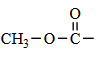• B .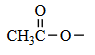• C .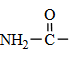• D .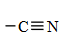38、

• A . D-甘露糖
• B . D-半乳糖
• C . D-核糖
• D . L-葡萄糖

39、

• A . 油脂
• B . 鞘磷脂
• C . 卵磷脂
• D . 脑磷脂

40、
Claisen酯缩合反应经常用来制备（）。
• A . β-羟基酯
• B . γ-羟基酯
• C . δ-羟基酯
• D . β-酮酸酯

41、

• A . 乙醚
• B . 正丁醇
• C . 正戊烷
• D . 氯乙烷

42、

• A .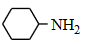• B .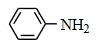• C .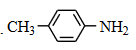• D .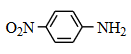43、

• A .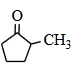• B .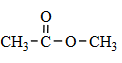• C .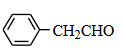• D .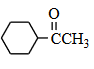44、

• A .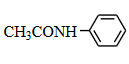• B .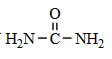• C .• D .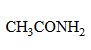45、

• A .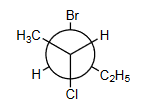• B .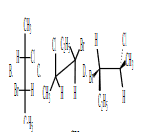• C .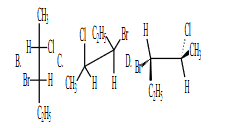46、

• A . （1）（2）（3）（4）
• B . （1）（3）（5）（4）
• C . （1）（2）（6）（4）
• D . （1）（3）（4）（6）

47、

• A .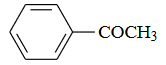• B .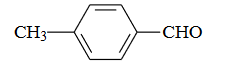• C . CH3CHO

48、

• A . 2－甲基－1－丁烯
• B . 3－甲基－2－戊烯
• C . 2－甲基－2－丁烯

49、

• A . ①
• B . ②
• C . ③
• D . ④
• E . ⑤

50、

• A . 乙酐
• B . 乙酰氯
• C . 乙酰胺
• D . 乙酸乙酯
• E . 乙酸

51、

• A . 淀粉
• B . 纤维素
• C . 麦芽糖
• D . 蔗糖
• E . 乳糖

52、

• A . 草酸
• B . 苯甲酸
• C . 蚁酸
• D . 硬脂酸
• E . 软脂酸

53、

• A . 烷烃
• B . 桥环烃
• C . 单环环烷烃
• D . 螺环烃
• E . 多环芳烃

54、

• A . 乙醛
• B . 乙醇
• C . 醋酸
• D . 石炭酸
• E . 水

55、

• A . 酸酐
• B . 酰胺
• C . 乙酸
• D . 卤代酸
• E . 醛

56、

• A . 乙酸乙酯
• B . 乙酰氯
• C . 乙酐
• D . 乙酰胺
• E . 乙醚

57、

• A . Na和FeCl3
• B . Na和Cu(OH)2
• C . Cu粉和FeCl3
• D . 溴水和FeCl3
• E . Na和AgNO3

58、

• A . 胱氨酸
• B . 丙氨酸
• C . α－氨基乙酸
• D . 组氨酸
• E . 缬氨酸

59、

• A . 甲胺
• B . 二甲胺
• C . 三甲胺
• D . 乙胺
• E . 二乙胺

60、

• A . 3
• B . 4
• C . 5
• D . 6
• E . 7

61、

• A . 甲醇
• B . 2-丙醇
• C . 乙醇
• D . 2-甲基-2-丙醇
• E . 1-丙醇